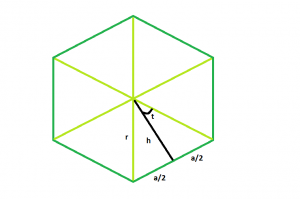# Area of a n-sided regular polygon with given Radius

Given a regular polygon of N sides with radius(distance from the center to any vertex) R. The task is to find the area of the polygon.

Examples:

```Input : r = 9, N = 6
Output : 210.444

Input : r = 8, N = 7
Output : 232.571
```

## Recommended: Please try your approach on {IDE} first, before moving on to the solution.In the figure, we see that the polygon can be divided into N equal triangles.

Looking into one of the triangles, we see that the whole angle at the centre can be divided into = 360/N parts.

So, angle t = 180/N.

Looking into one of the triangles, we see,

```h = rcost
a = rsint
```

We know,

```area of the triangle = (base * height)/2
= r2sin(t)cos(t)
= r2*sin(2t)/2
```

So, area of the polygon:

```A = n * (area of one triangle)
= n*r2*sin(2t)/2
= n*r2*sin(360/n)/2
```

Below is the implementation of the above approach:

## C++

 `// C++ Program to find the area ` `// of a regular polygon with given radius ` ` `  `#include ` `using` `namespace` `std; ` ` `  `// Function to find the area ` `// of a regular polygon ` `float` `polyarea(``float` `n, ``float` `r) ` `{ ` `    ``// Side and radius cannot be negative ` `    ``if` `(r < 0 && n < 0) ` `        ``return` `-1; ` ` `  `    ``// Area ` `    ``// degree converted to radians ` `    ``float` `A = ((r * r * n) * ``sin``((360 / n) * 3.14159 / 180)) / 2; ` ` `  `    ``return` `A; ` `} ` ` `  `// Driver code ` `int` `main() ` `{ ` `    ``float` `r = 9, n = 6; ` ` `  `    ``cout << polyarea(n, r) << endl; ` ` `  `    ``return` `0; ` `} `

## Java

 `// Java Program to find the area ` `// of a regular polygon with given radius ` ` `  `import` `java.util.*; ` `class` `GFG ` `{ ` `    ``// Function to find the area ` `    ``// of a regular polygon ` `    ``static` `double` `polyarea(``double` `n, ``double` `r) ` `    ``{ ` `        ``// Side and radius cannot be negative ` `        ``if` `(r < ``0` `&& n < ``0``) ` `            ``return` `-``1``; ` `     `  `        ``// Area ` `        ``// degree converted to radians ` `        ``double` `A = ((r * r * n) * Math.sin((``360` `/ n) * ``3.14159` `/ ``180``)) / ``2``; ` `     `  `        ``return` `A; ` `    ``} ` `     `  `    ``// Driver code ` `    ``public` `static` `void` `main(String []args) ` `    ``{ ` `        ``float` `r = ``9``, n = ``6``; ` `     `  `        ``System.out.println(polyarea(n, r)); ` `     `  `         `  `    ``} ` `} ` ` `  `// This code is contributed ` `// By ihritik (Hritik Raj) `

## Python3

 `# Python3 Program to find the area  ` `# of a regular polygon with given radius  ` ` `  `# form math lib import sin function ` `from` `math ``import` `sin ` ` `  `# Function to find the area  ` `# of a regular polygon  ` `def` `polyarea(n, r) : ` `     `  `    ``# Side and radius cannot be negative  ` `    ``if` `(r < ``0` `and` `n < ``0``) : ` `        ``return` `-``1` ` `  `    ``# Area  ` `    ``# degree converted to radians  ` `    ``A ``=` `(((r ``*` `r ``*` `n) ``*` `sin((``360` `/` `n) ``*`  `                 ``3.14159` `/` `180``)) ``/` `2``);  ` ` `  `    ``return` `round``(A, ``3``) ` ` `  `# Driver code  ` `if` `__name__ ``=``=` `"__main__"` `:  ` ` `  `    ``r, n ``=` `9``, ``6` `    ``print``(polyarea(n, r)) ` ` `  `# This code is contributed by Ryuga `

## C#

 `// C# Program to find the area ` `// of a regular polygon with given radius ` ` `  `using` `System; ` `class` `GFG ` `{ ` `    ``// Function to find the area ` `    ``// of a regular polygon ` `    ``static` `double` `polyarea(``double` `n, ``double` `r) ` `    ``{ ` `        ``// Side and radius cannot be negative ` `        ``if` `(r < 0 && n < 0) ` `            ``return` `-1; ` `     `  `        ``// Area ` `        ``// degree converted to radians ` `        ``double` `A = ((r * r * n) * Math.Sin((360 / n) * 3.14159 / 180)) / 2; ` `     `  `        ``return` `A; ` `    ``} ` `     `  `    ``// Driver code ` `    ``public` `static` `void` `Main() ` `    ``{ ` `        ``float` `r = 9, n = 6; ` `     `  `        ``Console.WriteLine(polyarea(n, r)); ` `     `  `         `  `    ``} ` `} ` ` `  `// This code is contributed ` `// By ihritik (Hritik Raj) `

## PHP

 ` `

Output:

```210.444
```

My Personal Notes arrow_drop_upCheck out this Author's contributed articles.

If you like GeeksforGeeks and would like to contribute, you can also write an article using contribute.geeksforgeeks.org or mail your article to contribute@geeksforgeeks.org. See your article appearing on the GeeksforGeeks main page and help other Geeks.

Please Improve this article if you find anything incorrect by clicking on the "Improve Article" button below.